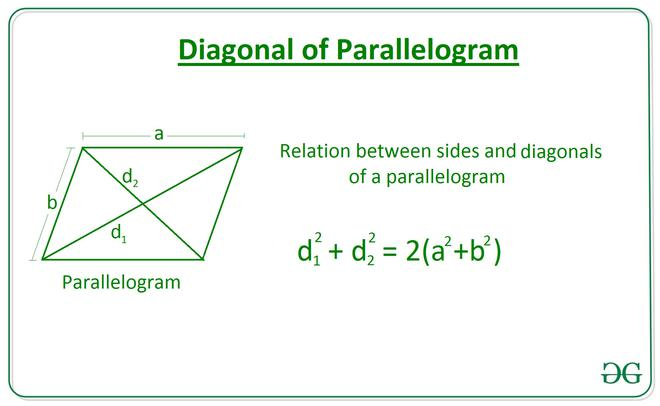# Length of a Diagonal of a Parallelogram using the length of Sides and the other Diagonal

• Last Updated : 17 Mar, 2021

Given two integers A and B, denoting the length of a parallelogram and an integer D, denoting the length of a diagonal, the task is to find the length of another diagonal of the parallelogram.

Examples:

Input: A = 10, B = 30, D = 20
Output: 40.0

Input: A = 6, B = 8, D = 10
Output: 10.0

Approach:
The relation between sides and diagonals of a parallelogram length of diagonal is given by the equation:Below is the implementation of the above approach:

## C++

 // C++ Program to implement// the above approach#include using namespace std; // Function to calculate the length// of the diagonal of a parallelogram// using two sides and other diagonalfloat Length_Diagonal(int a, int b, int d){     float diagonal = sqrt(2 * ((a * a) +                               (b * b)) - (d * d));     return diagonal;} // Driver Codeint main(){    int A = 10;    int B = 30;    int D = 20;     // Function Call    float ans = Length_Diagonal(A, B, D);     // Print the final answer    printf("%0.1f", ans);    return 0;} // This code is contributed by Rohit_ranjan

## Java

 // Java Program to implement// the above approachclass GFG{ // Function to calculate the length// of the diagonal of a parallelogram// using two sides and other diagonalstatic float Length_Diagonal(int a, int b, int d){     float diagonal = (float) Math.sqrt(2 * ((a * a) +                                 (b * b)) - (d * d));     return diagonal;} // Driver Codepublic static void main(String[] args){    int A = 10;    int B = 30;    int D = 20;     // Function Call    float ans = Length_Diagonal(A, B, D);     // Print the final answer    System.out.printf("%.1f", ans);}} // This code is contributed by Rajput-Ji

## Python

 # Python Program to implement# the above approach import math # Function to calculate the length# of the diagonal of a parallelogram# using two sides and other diagonaldef Length_Diagonal(a, b, d):     diagonal = math.sqrt(2 * ((a**2) \    + (b**2)) - (d**2))     return diagonal  # Driver CodeA = 10B = 30D = 20 # Function Callans = Length_Diagonal(A, B, D) # Print the final answerprint(round(ans, 2))

## C#

 // C# Program to implement// the above approachusing System;class GFG{ // Function to calculate the length// of the diagonal of a parallelogram// using two sides and other diagonalstatic float Length_Diagonal(int a, int b, int d){     float diagonal = (float) Math.Sqrt(2 * ((a * a) +                                 (b * b)) - (d * d));     return diagonal;} // Driver Codepublic static void Main(String[] args){    int A = 10;    int B = 30;    int D = 20;     // Function Call    float ans = Length_Diagonal(A, B, D);     // Print the readonly answer    Console.Write("{0:F1}", ans);}} // This code is contributed by Rajput-Ji

## Javascript

 
Output:
40.0

Time Complexity: O(1)
Auxiliary Space: O(1)

My Personal Notes arrow_drop_up